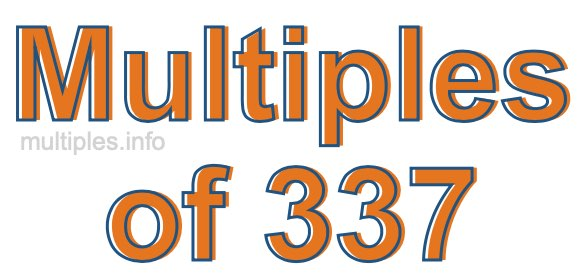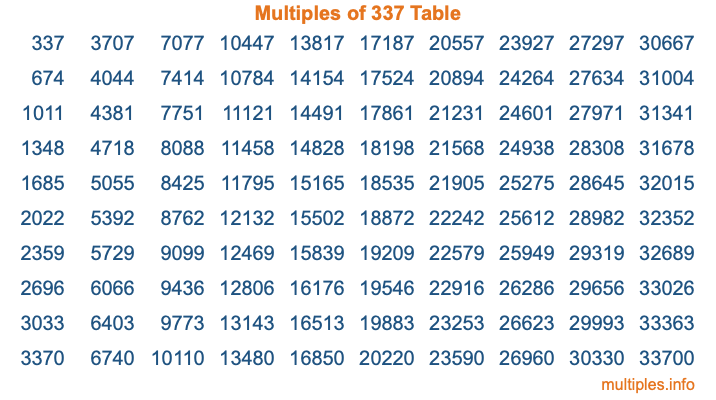Multiples of 337Welcome to the Multiples of 337 page. Here we will first teach you everything you will ever need to know about the multiples of 337, and then give you a study guide summary of everything we taught you to make sure you remember it all. Use this page to look up facts and learn information about the multiples of 337. This page will make you a multiples of three hundred thirty-seven expert!

Definition of Multiples of 337
Multiples of 337 are all the numbers that when divided by 337 equal an integer. Each of the multiples of 337 are called a multiple. A multiple of 337 is created by multiplying 337 by an integer.

Therefore, to create a list of multiples of 337, you start with 1 multiplied by 337, then 2 multiplied by 337, then 3 multiplied by 337, and so on for as long as you want. Thus, the list of the first five multiples of 337 is 337, 674, 1011, 1348, and 1685. To see a larger list of multiples of 337, see the printable image of Multiples of 337 further down on this page. We also have a category where you can choose any nth multiple of 337.

Multiples of 337 Checker
The Multiples of 337 Checker below checks to see if any number of your choice is a multiple of 337. In other words, it checks to see if there is any number (integer) that when multiplied by 337 will equal your number. To do that, we divide your number by 337. If the the quotient is an integer, then your number is a multiple of 337.

Is  a multiple of 337?

Least Common Multiple of 337 and ...
A Least Common Multiple (LCM) is the lowest multiple that two or more numbers have in common. This is also called the smallest common multiple or lowest common multiple and is useful to know when you are adding our subtracting fractions. Enter one or more numbers below (337 is already entered) to find the LCM.

Check out our LCM Calculator if you need more details about the Least Common Multiple or if you need the LCM for different numbers for adding and subtraction fractions.

nth Multiple of 337
As we stated above, 337 is the first multiple of 337, 674 is the second multiple of 337, 1011 is the third multiple of 337, and so on. Enter a number below to find the nth multiple of 337.

th multiple of 337

Multiples of 337 vs Factors of 337
337 is a multiple of 337 and a factor of 337, but that is where the similarities end. All postive multiples of 337 are 337 or greater than 337. All positive factors of 337 are 337 or less than 337.

Below is the beginning list of multiples of 337 and the factors of 337 so you can compare:

Multiples of 337: 337, 674, 1011, 1348, 1685, etc.

Factors of 337: 1, 337

As you can see, the multiples of 337 are all the numbers that you can divide by 337 to get a whole number. The factors of 337, on the other hand, are all the whole numbers that you can multiply by another whole number to get 337.

It's also interesting to note that if a number (x) is a factor of 337, then 337 will also be a multiple of that number (x).

Multiples of 337 vs Divisors of 337
The divisors of 337 are all the integers that 337 can be divided by evenly. Below is a list of the divisors of 337.

Divisors of 337: 1, 337

The interesting thing to note here is that if you take any multiple of 337 and divide it by a divisor of 337, you will see that the quotient is an integer.

Multiples of 337 Table
Below is an image of the first 100 multiples of 337 in a table. The table is in chronological order, column by column. The first column has the first ten multiples of 337, the second column has the next ten multiples of 337, and so on.The Multiples of 337 Table is also referred to as the 337 Times Table or Times Table of 337. You are welcome to print out our table for your studies.

Negative Multiples of 337
Although not often discussed or needed in math, it is worth mentioning that you can make a list of negative multiples of 337 by multiplying 337 by -1, then by -2, then by -3, and so on, to get the following list of negative multiples of 337:

-337, -674, -1011, -1348, -1685, etc.

Multiples of 337 Summary
Below is a summary of important Multiples of 337 facts that we have discussed on this page. To retain the knowledge on this page, we recommend that you read through the summary and explain to yourself or a study partner why they hold true.

There are an infinite number of multiples of 337.

A multiple of 337 divided by 337 will equal a whole number.

337 divided by a factor of 337 equals a divisor of 337.

The nth multiple of 337 is n times 337.

The largest factor of 337 is equal to the first positive multiple of 337.

337 is a multiple of every factor of 337.

337 is a multiple of 337.

A multiple of 337 divided by a divisor of 337 equals an integer.

337 divided by a divisor of 337 equals a factor of 337.

Any integer times 337 will equal a multiple of 337.

Multiples of a Number
Here you can get the multiples of another number, all with the same attention to detail as we did for multiples of 337 on this page.

Multiples of
Multiples of 338
Did you find our page about multiples of three hundred thirty-seven educational? Do you want more knowledge? Check out the multiples of the next number on our list!